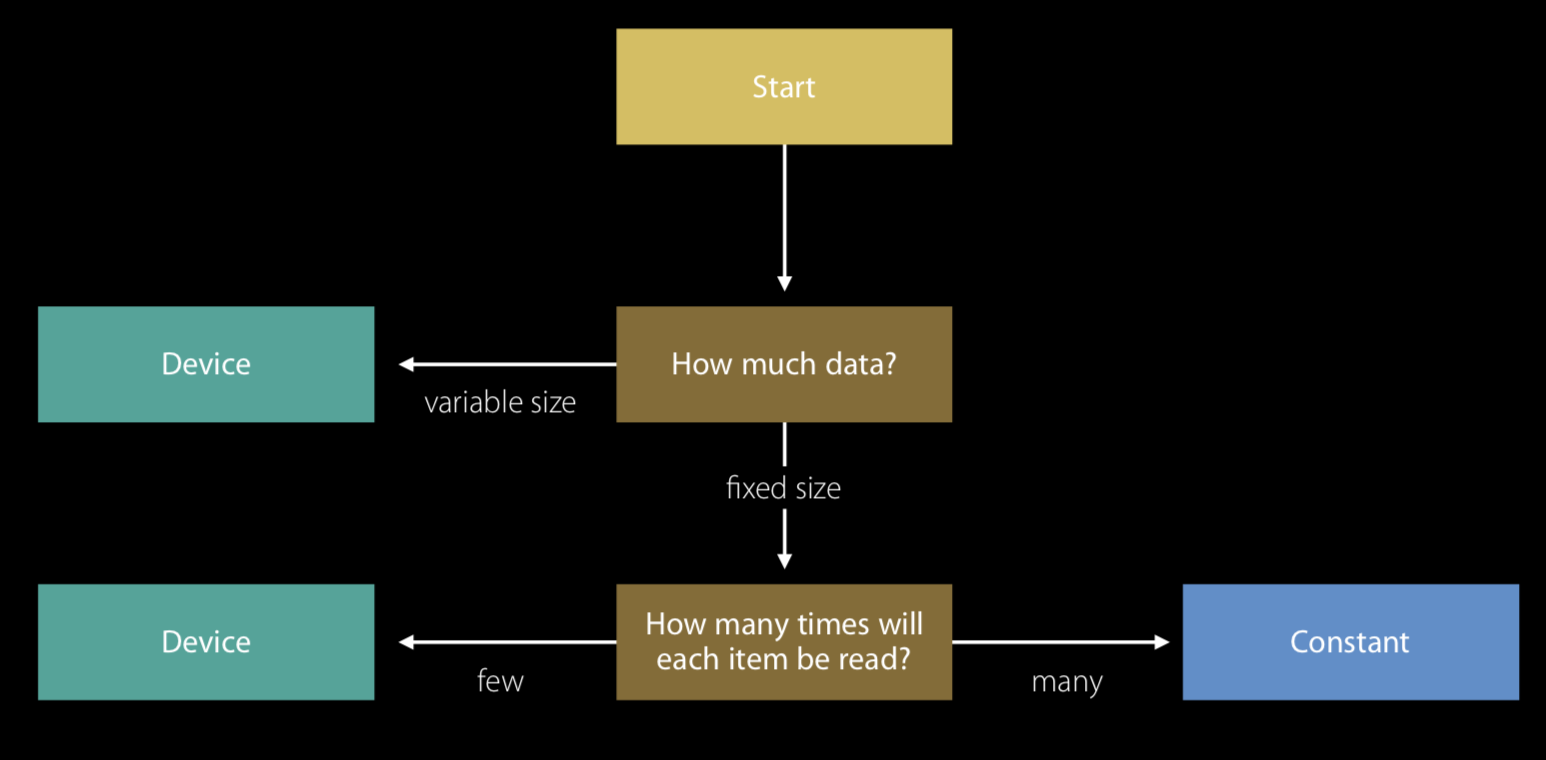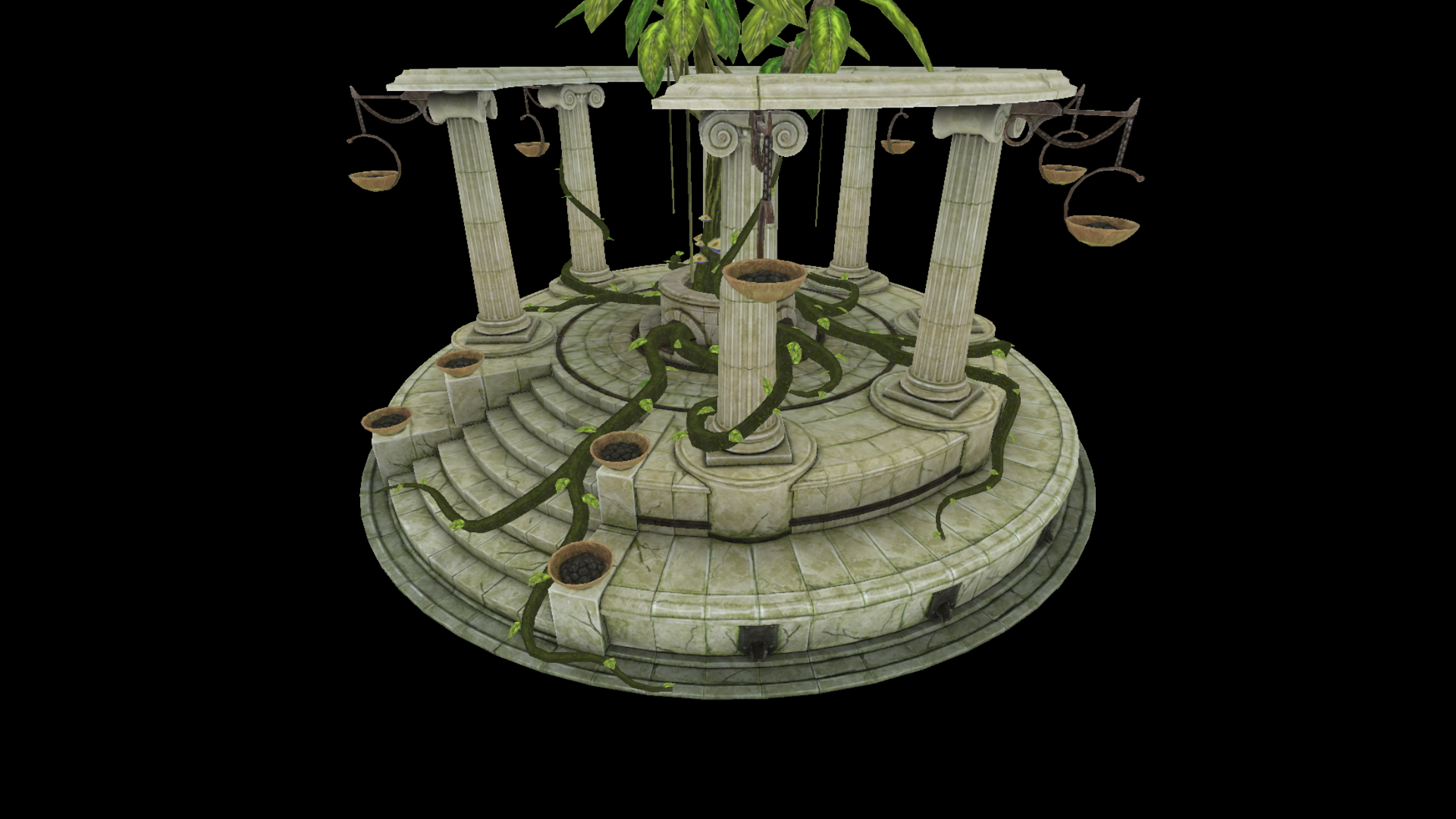# 【一步步学Metal图形引擎5】-《Uniform Buffer》

## 一、Uniform Buffer的作用

Uniform Buffer和OpenGL中的一致变量类似，用来定义和传递渲染过程中的一些常量数据，例如：坐标系变换矩阵数据、光照参数以及其他自定义常量数据。Metal中通过一个专门的MTLBuffer来保存和传递这些常量数据，这个buffer就成为Uniform Buffer，buffer中的数据通过一个结构体来定义和组织。

GPU访问内存的方式有多种，不同的地址空间内存数据的访问模式和内存分配方式不同，Metal中向开发者提供了明确定义内存访问模式的语法属性，用来明确声明buffer数据内存分配的区域。开发者要根据数据使用的情景和需求，合理的定义数据的内存分配方式，从而正确高效的实现GPU数据的读写。

Metal中地址空间属性描述主要有以下几种：

• device
• constant

### device和constant如何选择

• device：内存大小灵活可变，内存大小没有限制，可读写，数据访问没有constant高效；
• constant：内存大小固定，只读，数据访问优化高效。## 二、源码分析

typedef struct
{
matrix_float4x4 projectionMatrix;
matrix_float4x4 modelViewMatrix;
} Uniforms;


### Render.m

id <MTLBuffer> _uniformBuffer;


    const MTLResourceOptions storageMode = MTLResourceStorageModeShared;
_uniformBuffer = [_device newBufferWithLength:sizeof(Uniforms)
options:storageMode];


- (void) mtkView:(nonnull MTKView *)view drawableSizeWillChange:(CGSize)size
{
float aspect = size.width / (float)size.height;
float _fov = 65.0f * (M_PI / 180.0f);
float _nearPlane = 1.0f;
float _farPlane = 1500.0f;
_projectionMatrix = matrix_perspective_left_hand(_fov, aspect, _nearPlane, _farPlane);
}


/// Update app state for the current frame.
- (void)updateGameState
{
Uniforms * uniforms = (Uniforms*)_uniformBuffer.contents;
uniforms->projectionMatrix = _projectionMatrix;
matrix_float4x4 viewMatrix = matrix_multiply(matrix4x4_translation(0.0, 0, 1000.5),
matrix_multiply(matrix4x4_rotation(-0.5, (vector_float3){1,0,0}),
matrix4x4_rotation(_rotation, (vector_float3){0,1,0} )));
vector_float3 rotationAxis = {0, 1, 0};
matrix_float4x4 modelMatrix = matrix4x4_rotation(0, rotationAxis);
matrix_float4x4 translation = matrix4x4_translation(0.0, 0, 0);
modelMatrix = matrix_multiply(modelMatrix, translation);

uniforms->modelViewMatrix = matrix_multiply(viewMatrix, modelMatrix);

_rotation += 0.002f;
}


//...
[renderEncoder setVertexBuffer:_uniformBuffer offset:0 atIndex:1];
//...


vertex ColorInOut vertexShader(Vertex in [[ stage_in ]],
constant Uniforms & uniforms [[ buffer(1) ]])
{
ColorInOut out;
float4 position = float4(in.position, 1.0);
out.position = uniforms.projectionMatrix * uniforms.modelViewMatrix * position;
out.texCoord = in.texCoord;
return out;
}


## 四、运行效果10-016354
01-2295004-261311
05-2068
02-271078
03-20429
03-31252
04-04378
03-31202点击重新获取扫码支付余额充值